Trends in Periodic Properties- 2

# Trends in Periodic Properties- 2 Notes | Study Inorganic Chemistry - Chemistry

## Document Description: Trends in Periodic Properties- 2 for Chemistry 2022 is part of Inorganic Chemistry preparation. The notes and questions for Trends in Periodic Properties- 2 have been prepared according to the Chemistry exam syllabus. Information about Trends in Periodic Properties- 2 covers topics like and Trends in Periodic Properties- 2 Example, for Chemistry 2022 Exam. Find important definitions, questions, notes, meanings, examples, exercises and tests below for Trends in Periodic Properties- 2.

Introduction of Trends in Periodic Properties- 2 in English is available as part of our Inorganic Chemistry for Chemistry & Trends in Periodic Properties- 2 in Hindi for Inorganic Chemistry course. Download more important topics related with notes, lectures and mock test series for Chemistry Exam by signing up for free. Chemistry: Trends in Periodic Properties- 2 Notes | Study Inorganic Chemistry - Chemistry
 1 Crore+ students have signed up on EduRev. Have you?

Electron  Affinity

Amount of energy released when an electron is added to an isolated gaseous atom.
x( g ) + e- → x- ( g ) + E.A.
Units : k J mol-1 ,  k Cal mol-1 and  eV per atom.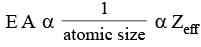Cl  has  the  highest  E.A.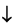• EA measures the tightness with which an atom can hold the additional e- .
• Larger value of E.A. reflects the greater tendency of an atom to accept the e- .

Electron gain Enthalpy When expressed in terms of enthalpy change (ΔH) then it is termed as E.G.E. Remember that
ΔH = - ve for exothermic change.

For EA1, energy is released              ∴ΔHEA1 = - ve

For EA2, onwards is required         ∴ ΔHEA2 = + ve

EA1 + EA2                                               ∵ ΔH = +ve

Factors affecting Electron Affinity

(i) Nuclear Charge: Varies directly As nuclear charge increases, the incoming e- is more strongly attracted and more amount of energy is liberated.

(ii) Atomic Size: Varies inversely

• As atomic size increases, The distance between nucleus and incoming electron also increases. The e- is less stabilized.

Less amount of energy is liberated.

So, larger atoms have lower E.A. value

(iii) E.C.: Stable electronic configurations have less tenoency to accept electron, so they have lower E.A. values.

Periodic Trends
(i) In a Period :
Along a period, electron affinity increases [with exception] as Zeff ­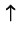.

• X have highest E.A. : In corresponding periods (Halogens). By accepting 1e-, achieve ns2p6 configuration.
• Noble gase have zero E.A. :As they have stable configuration, so they do not have any tendency to accept e.
• E.A., Be, Mg → EA almost zero : They have all paired e-s. So they have stable configuration.
• E.A. of N, P → extremely low : These have stable half filled E.C. Their p orbitals one half filled in their valence shells.

(ii) In a group : Along a group, electron affinity decreases after 3rd period. Between 2nd and 3rd period in p block electron affinity of 2nd period is lesser due to high electron density.

F = 328,  Cl = 349,  Br =324,  I = 295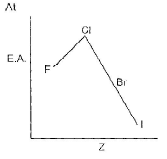Exception

(1) A fully filled and half filled which have low values or even sometimes energy is required rather than getting released.

(2) 2nd period has lower value than 3rd owing to repulsion between electrons.

Unexpected E.A.: EA of chlorine is higher than that of F.
In general: The third period elements have higher EA values than 2nd period elements.

Electronegativity (E.N.)

• It is defined as, the tendency of an atom to attract the shared pair of electron in a molecule.

Factor affecting electronegativity

(1) Nuclear attraction : varies directly
(2) Atomic radius : varies inversely
(3) Change on ions : More positive charge more electronegativity and more –ve change less electronegativity. (4) Hybridisation : to be discussed later in bonding.
⇒ When ENC increases more EN, also increases.

Periodic Trends

Across the Period : The E.N. values increase along a period from left to right.
Down the Group:

• The E.N. values decrease down the group.

Most Electronegative Atom of P.T.:

• The Black Sheep is (F).
• Least EN. atom → (Fr)
• 1st Group elements (Alkali metals) have lowest EN. in a period.
• The Halogens are most electronegative in a period.
• Non metals have high EN. values.

Scale of measurement

(1) Pauling’s Scale (1932):

• Pauling assigned an arbitrary EN value of 4.0 to the most electronegative atom F.
• The E.N. of other elements were as by keeping this value as the standard reference.
• The E.N. difference between two atoms is determined by the expression,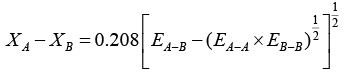XA = E.N. of A

XB = E.N. of B.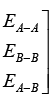Bond energies of A - A, B - B, A - B molecules.

(2) Mulliken Scale (1934) : According to this scale, EN. of an atom is the average sum of I E. and EA. of an atom.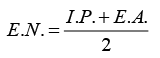In Pauling Scale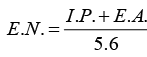(If I.P., E.A. are taken in eV)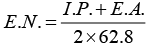(I.P., E.A. = Kcal mol-1)

Factors dependent on electronegativity

(1) % ionic character varies directly.

(2) Strength of bond varies directly.

Application of E.N.

(1) Polarity of a molecule : As the DE increases between two bonded atoms; it polarity also increases.
Eg. H - I < HBr < H - Cl < H - F .....dipole moment The direction of dipole is also determine by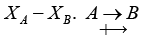The more electronegative atom constitutes the negative pole.

(2) Nature of the bond of a molecule

If the Δ E.N. is less (< 1 .7) the bond the has more covalent character..
If the Δ E.N is more (> 1.7) the bond the has more ionic character..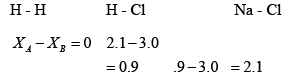Allred Scale for measurement of % ionic character  of a covalent bond : % ionic character of a bond =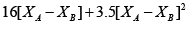(3) Determination of Bond Strength: As the ΔE = X A - X B increases, a stronger covalent bond is formed.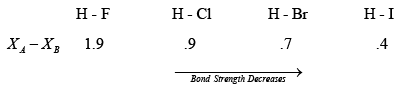Table : Hydrides MHx - E.N. of M and Nature

 S.NO. Δ E.N .(M .H .) Nature Eg. 1. M < H Basic LiH < NaH < KH < RbH < CsH BeH2 < MgH2 < CaH2 < SrH2 < BaH2 < RaH2 AlH3 < GaH3 < InH3 < TiH3 NH3 < PH3 < AsH3 < SbH3 < BiH3 Transition metal hydrides 2. M = H Neutral CH4 < SiH4 < GeH4 < SnH4 < PhH4 3. M > H Acidic H2O < H2S < H2Se < H2Te < H2PO HF < HCl < HBr < HI

INERT PAIR EFFECT
Inert pair effect term refers to the resistances of a pair of s electrons to be lost or to participate in covalent bond formation.
Example:

(1) Group III A :

(A) TI(3+): 2, 8; 18, 32, 18

Tl(+) : 2,8, 18, 32, 18, 2

Tl(1) compounds are more stable than Tl(III) compounds.

(B) The mean bond energy for chlorides are GaCl= 242, InCl3 = 206 and TlCl3 = 153 kJ mol-1.
Thus the s electrons are most likely to be Inert in thallium and TIClis the least stable among these chlorides.

(2) Group IVA:

(A) Sn2+ : 2, 8, 18, 18, 2 (outer 5s2 electrons not lost)

Sn4+: 2, 8, 18, 18

Sn(II) exist as a simple ion Sn2+ and is a strong reducing agent whereas Sn(IV) is covalent.

(B) Pb2 : 2. 8, 18, 32. 18, 2 (outer 6selectrons not lost)

Pb4: 2,8, 18, 32, 18

Pb2 is Ionic, stable and more common than Pb (IV) which is oxidizing.

(3) GroupVA:

(A) Arsenic and atomic show a variable valence of 3 and 5 while Bismuth is only trivalent.
Bi3+ : 2, 8, 18, 32, 18, 2 (outer 6s2 electron not lost)

Bi3+ is stable and Sb3+ is a reducing agent.

The document Trends in Periodic Properties- 2 Notes | Study Inorganic Chemistry - Chemistry is a part of the Chemistry Course Inorganic Chemistry.
All you need of Chemistry at this link: Chemistry

## Inorganic Chemistry

36 videos|71 docs|16 tests
 Use Code STAYHOME200 and get INR 200 additional OFF

## Inorganic Chemistry

36 videos|71 docs|16 tests

Track your progress, build streaks, highlight & save important lessons and more!

,

,

,

,

,

,

,

,

,

,

,

,

,

,

,

,

,

,

,

,

,

;